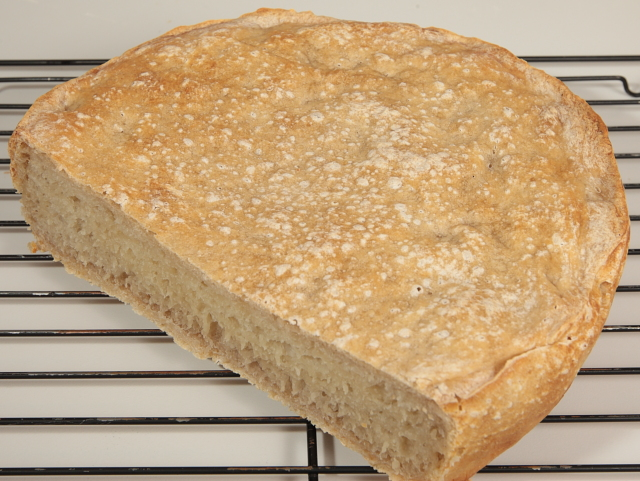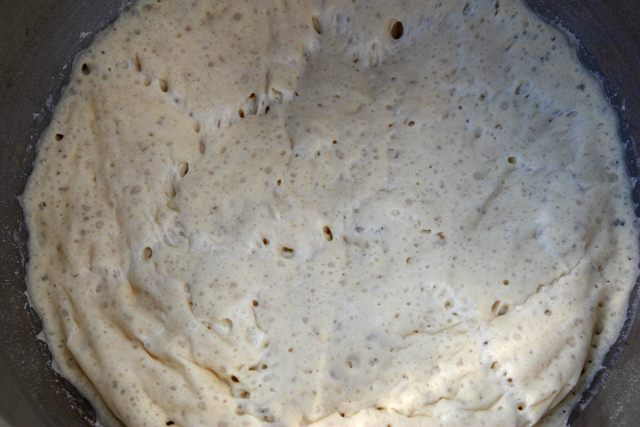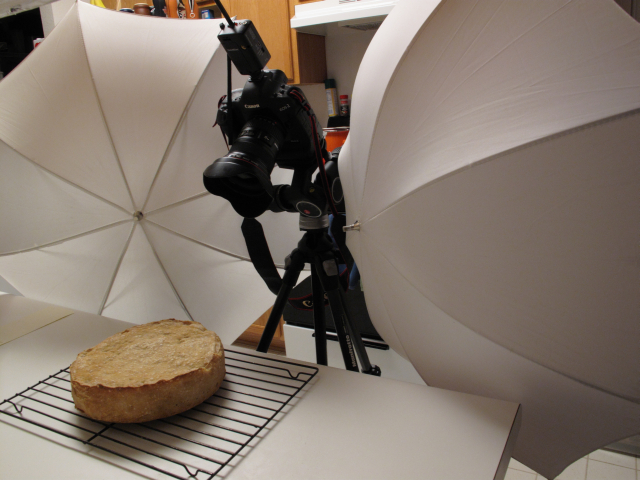# A second attempt at breadI had another go at experimenting with bread. And ended up with a loaf that looked like a cake!I tried taking this picture outside. But the stainless steel bowl was creating a crescent shaped blown out area. So I moved it into the shade. However, the colors were slightly off. There was a bluish tint in the shadows.For the cake bread, I used clamshell lighting. Two light stands pressed as close to the camera and food as possible. At an eight power, I was able to get f/11 at ISO 200. The only weirdness was that one flash was slaved over Canon’s IR. This halved my sync speed from 1/250 to 1/125. I would have thought that I could have at least seen one of the flashes light the picture. Maybe the IR communication parted needed extra time to complete. But the picture was black at 1/250 and lit at 1/125.

Recipe follows:

Weigh an amount of starter into the bowl. For example, the starter weighed 200 grams. Now, figure out the water and flour components in that starter with the following equation:

w=200*132/(132+96), f=200*(1-132/(132+96))
w=116g, f=84g

Baker’s percentages compare one ingredient to another. In the case of bread, it compares water to flour. The weight of the flour is defined to be 100%. The weight of the water is then
compared to the flour weight. Given the above water and flour weights, the Baker’s percentage comes out to be:

w=136.6% f=100%

The hydration of the dough is the Baker’s percentage of water. In the above example, we have a hydration level of 136.6%

Now, we want to add more flour (we don’t want a tiny loaf) and we want to bring the hydration level down to 85%. Weigh the amount of flour that we have added to the bowl. For example,
if I added 300 grams more flour, then

w=116g, f=(300+84)=384g

At 85% of the flour weight, the final water weight should be

w=384*0.85=326

How much water should we add to get there?

w=(x+116)=326

Solving for x, then

x=210

Now we have the following water and flour components

w=326g, f=384g

Let the bowl proof in a warm environment for six hours. In my oven with the light on, the temperature in Fahrenheit is 90. We are looking to create more yeast and more acid (lactic and acetic).

Next, we need to add salt at a Baker’s percentage of 0.02%. But, first, we must figure out how much flour to add to bring the mixture down to a final hydration level of 70%

326=(x+384)*0.7
x=326/0.7-384
x=82g

Now that we know the final flour weight of:

f=384+82
f=466g

We can calculate how much salt to add:

s=466*0.02
s=9g

Mix well to incorporate the salt evenly.

Cut a 29″x7″ rectangle of parchment paper create a tube by stapling the top. Use the rest of the parchment paper to line the bottom thoroughly.